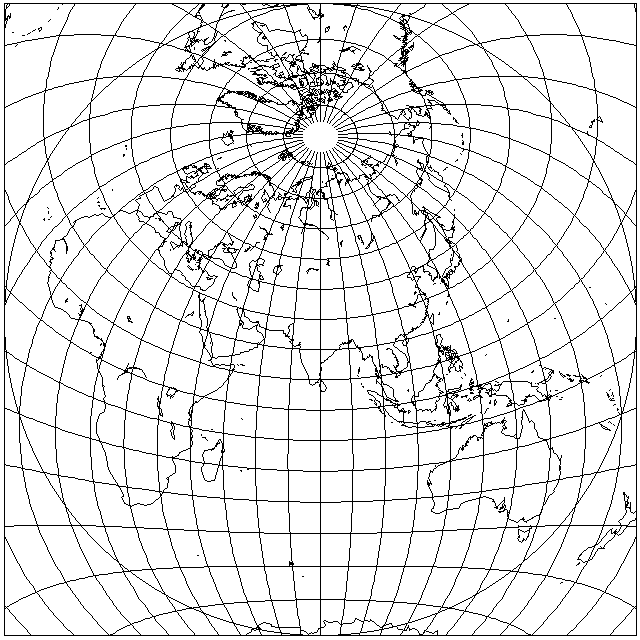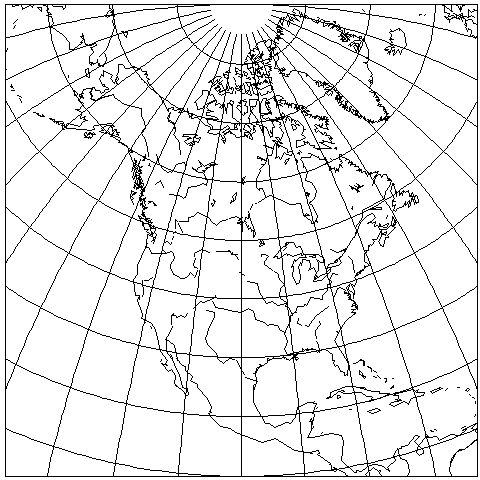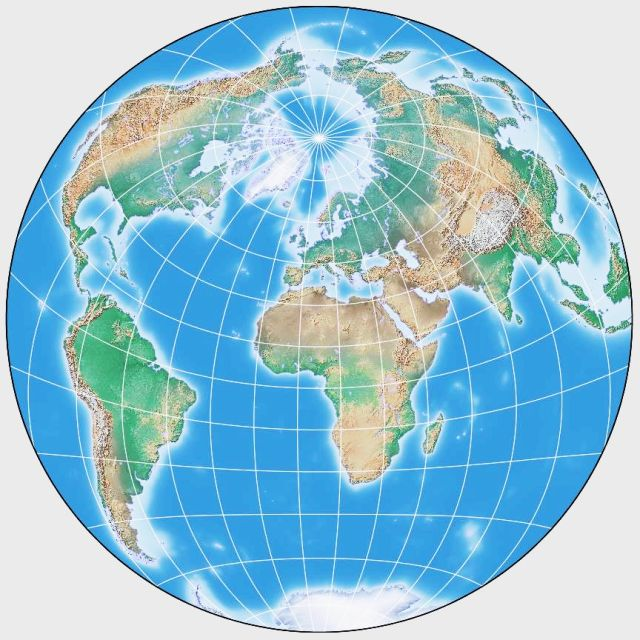[Next] [Up] [Previous]

# Airy's Minimum-Error Azimuthal Projection

This azimuthal projection is an analytic solution to the problem of minimizing shape and area error in a map projection:It was described by the British Astronomer Royal, George Biddell Airy, in an 1861 paper.

Such a solution is possible when the area to be depicted is a circle on the globe, since then the symmetry involved means that the resulting projection must be azimuthal. For maps of the whole world, only approximate polynomial projections have been found through numerical methods.

The area over which error was minimized was the circle inscribed in the square boundary of the map above.

What sort of error is being minimized in this projection?

The fundamental theory of error in map projections is based on Tissot's Indicatrix. At each point on the map, an infinitesimal circle on the globe will be mapped to an infinitesimal ellipse. Scale up that ellipse to finite size, by dividing by the size of the infinitesimal circle on the globe, and its semimajor axis is a, and its semiminor axis is b.

a and b can be determined as follows:

Let vlat (or S sub theta) be the scale along the meridian at a point on the map, and vlong (or S sub phi) be the scale along the parallel at that point, and let xang (or psi) be the angle at which the meridian and the parallel cross. These values can be fairly easily determined from the formula for the projection.

Then, a and b can be found from these equations:

```         _________________________________________________
/     2        2
big = \/  vlat  + vlong  + 2 * vlat * vlong * sin( xang )
_________________________________________________
/     2        2
small = \/  vlat  + vlong  - 2 * vlat * vlong * sin( xang )

big + small
a = -------------
2

big - small
b = -------------
2
```

The proportion of error in the area is simply:

```a * b - 1
```

The maximum angular deformation at a given point is:

```            /  a-b  \
2 * arcsin |  -----  |
\  a+b  /
```

and the quantity representing error in general, which is minimized by a minimum error projection, is:

```         2           2
( a - 1 ) + ( b - 1 )
```

Airy's minimum-error projection has the formula:

```r = cot(theta/2) * ln( 1/cos(theta/2) ) + tan(theta/2)*k
```

where

```                 2
k = ( cot(chi/2) )  * ln( 1/cos(chi/2) )
```

and chi is the angle away from the center of the projection over which errors are to be minimized, and theta is the angle away from the center of the projection of the point to be drawn (90 minus the latitude for a projection centered on the North Pole).

Of course, the illustration above was intended to show the projection working hard, by including a large expanse which would provide plenty of error to correct. But if the concern was to minimize error only over the land area, obviously error could be reduced further by interrupting the projection in the Indian Ocean; the existence of a minimum-error projection does not automatically minimize error if it does not match the shape of the area being portrayed.

For an example of a more reasonable use of this projection, here is a map of North America:However, as a less optimal projection, Clarke's Minimum-Error Perspective Projection, which we will see on the next page, was used with maps covering significantly more than a hemisphere, perhaps it isn't that inappropriate to use this projection even for a large area. Here is what it looks like when it is attempted to include North and South America, Asia, and Africa, all in one azimuthal projection:This map was drawn by G.Projector, using a source raster image from the Natural Earth website.

[Next] [Up] [Previous]